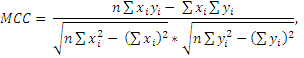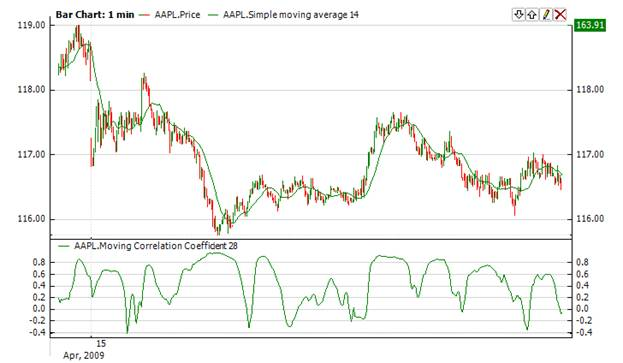﻿Moving Correlation Coefficient

# Moving Correlation Coefficient

The Mcc calculates a correlation coefficient of two data series over the last n periods. This statistical calculation is used to determine if two series of numbers are related. The closer the value is to 1, the closer the data is related.Calculationwhere n – is the period.Chart ExampleImplementation and Usage

To initialize Mcc indicator use one of the following constructors:

Mcc – sets default values: period = 14

Mcc(Int32) – sets value for period

Mcc(TimeSpan) – sets time period

Use

MCC - property to get current valueExample
C#
``` 1Calculate the correlation coefficient between simple moving average and price
2// Create new instance
3Mcc mcc = new Mcc(28);
4Sma sma = new Sma(14);
5